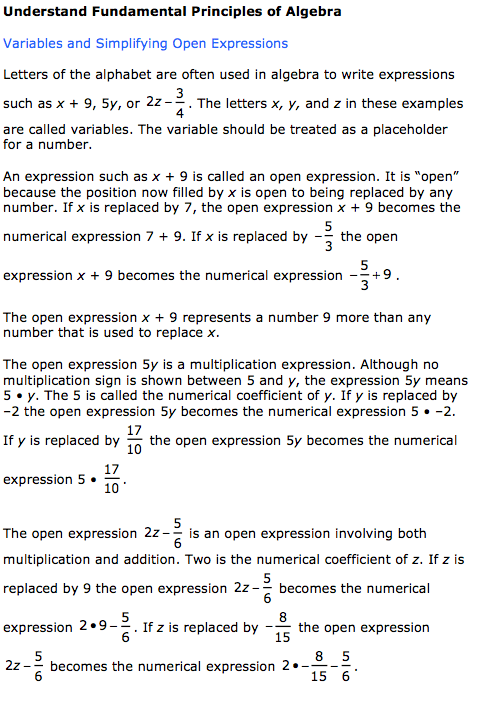Welcome, Student Name | Student Demo | Buy Now

 Contents Mathematics (190)Number Properties and OperationsPrinciples of AlgebraSimplifying ExpressionsSolving Equations:X + A = BSolving Equations:AX = BOne Variable EquationsLinear Word ProblemsInequalitiesLinear InequalitiesLinear Word Problems InequalitiesGraphing Equations and InequalitiesApplication ProblemsMeasurement and Geometry ConceptsProbability and StatisticsProblem Solving and ReasoningPractice Math Tests Main Menu Exit Student Demo Buy Now for \$39.95 and begin using the entire course in less than two minutes